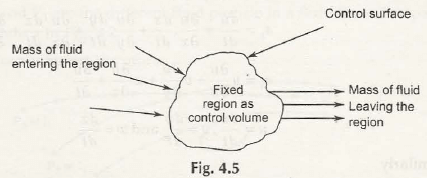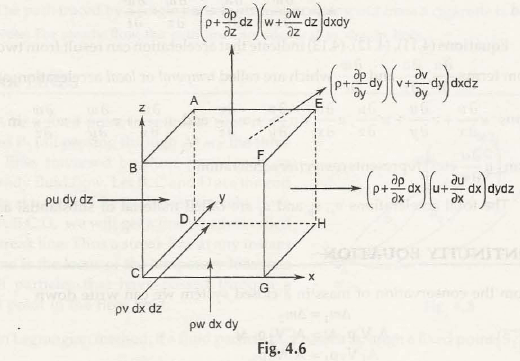To derive the continuity equation in three dimensions in differential form, we consider the concept of control volume as shown in Fig. 4.5Take an element of fluid from the control volume and control surface to create rectangular parallelepiped as shown in Fig. 4.6Let us consider mass is entering from ABCD surface and leave the body from EFGH in xdirection.

Rate of mass enters the fluid element = ρudydz

Rate of mass leaves the fluid element = (ρ + ∂ρ/∂x  dx )(u + ∂u/∂x  dx )dydz

= [ρ u + ρ ∂u/∂x  dx + u ∂ρ/∂x  dx +  ∂ρ/∂x.  ∂u/∂x 〖(dx)〗^2 ]dydz

= [ρ u +(∂(ρu))/∂x  dx] dydz    {∂ρ/∂x.  ∂u/∂x 〖(dx)〗^2  is neglected}
We know that,

For control volume:

Rate of mass entering control volume = Rate of mass leaves + Rate of accumulation of mass

Substituting the values, we get-

ρu dydz     =    [ρ u +(∂(ρu))/∂x  dx]dydz    +     Rate of accumulation of mass

Thus,
Rate of accumulation of mass = ρu dydz – [ρ u +(∂(ρu))/∂x  dx]dydz

= – (∂(ρu))/∂xdxdydz

Similarly,

Rate of accumulation of mass in y direction can be given by,

= – (∂(ρv))/∂ydxdydz

And,

Rate of accumulation of mass in z direction can be given by,

= – (∂(ρw))/∂zdxdydz

Adding all above equations of rate of accumulation of mass, we get the rate of accumulation of the element rectangular parallelepiped-

= –[(∂(ρu))/∂x  + (∂(ρv))/∂y  + (∂(ρw))/∂z]dxdydz

Or,

∂ρ/∂tdxdydz = –[(∂(ρu))/∂x  + (∂(ρv))/∂y  + (∂(ρw))/∂z]dxdydz

Or,

∂ρ/∂t= –[(∂(ρu))/∂x  + (∂(ρv))/∂y  + (∂(ρw))/∂z]

Or,

∂ρ/∂t + [(∂(ρu))/∂x  + (∂(ρv))/∂y  + (∂(ρw))/∂z] = 0            (General Continuity Equation)

Note:

The above general continuity equation is applicable to:

Uniform Flow and Non-uniform Flow

Then, continuity equation becomes

(∂(ρu))/∂x  +  (∂(ρv))/∂y  +  (∂(ρw))/∂z= 0

Compressible Flow and Incompressible Flow

For incompressible flow, ∂ρ/∂t= 0 andρ = constant

Then, continuity equation becomes

∂u/∂x  +  ∂v/∂y  +  ∂w/∂z= 0

If we consider the above equation in vector form, then it can be written as-

∂ρ/∂t + V ⃗. (ρV ⃗) = 0

Where,

V ⃗ = Velocity Vector = i ̂ ∂/∂x  +j ̂ ∂/∂y  +k ̂ ∂/∂z

Suppose the density ρ of liquid remains same with time that means ∂ρ/∂t= 0

Hence,

V ⃗. (ρV ⃗) = 0

If the density ρ = constant, then the above equation can be written as-

V ⃗. (V ⃗) = 0

Links of Next Mechanical Engineering Topics:-### Customer Reviews

My Homework Help
Rated 5.0 out of 5 based on 510 customer reviews at